# Ultra-Wideband (UWB) Planar Monopole Antennas

This example shows how to design UWB planar monopole antennas. Planar monopole antennas are simple in geometry and provide an ultrawideband (UWB) operating bandwidth. To create a planar monopole, replace a conventional wire monopole with planar elements in a variety of shapes to increase the surface area of the monopole. To design a planar monopole antenna, mount a planar metal plate on the ground plane. The ground plane can also have various shapes.

### Annular Planar Monopole Antenna

This figure shows the geometry and dimensions of an annular planar monopole antenna. The radiating element is an annular ring, with an outer radius `(rBig)` of `25 mm` and an inner radius `(rSmall)` of `10 mm`, located vertically above a square ground plane with a side length of `305 mm`.` `The feedgap (`d)`between the feedpoint in the radiator and the ground plane and the width of the feedstrip (`w) `are set to `0.8 mm` and `0.6 mm`, respectively, to enhance the impedance bandwidth.` `The offset value is set to `0.2 mm` to make a perfect connection between the feedstrip and the annular ring radiator.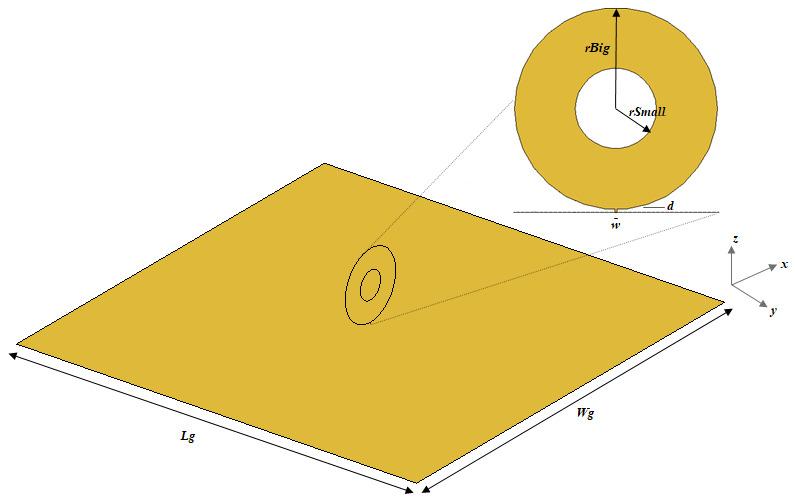#### Create Ground Plane

Create the square ground plane with a side length of `305 mm` using the `antenna.Rectangle` function.

```Lg = 305e-3; Wg = 305e-3; groundPlane = antenna.Rectangle("Length",Lg,"Width",Wg);```

Create the feedstrip using the `antenna.Rectangle` function. To create an outer and inner circle, use the `antenna.Circle` function. To create the annular ring radiator, subtract the `innerCircle` from the `outerCircle` and add in the `FeedStrip`.

```d = 0.8e-3; w = 0.6e-3; offset = 0.2e-3; % offset value when a vertex touches the feed. FeedStrip = antenna.Rectangle("Length",w,"Width",d,"Center",[0 d/2]); rBig = 25e-3; rSmall = 10e-3; outerCircle = antenna.Circle("Radius",rBig,"Center",[0 rBig+d-offset]); innerCircle = antenna.Circle("Radius",rSmall,"Center",[0 rBig+d-offset]); radiator = outerCircle-innerCircle+FeedStrip;```

#### Create Planar Monopole

To create the annular ring planar monopole antenna, use the `monopoleCustom` object.

```ant = monopoleCustom ("Radiator",radiator,"GroundPlane",groundPlane); show(ant)```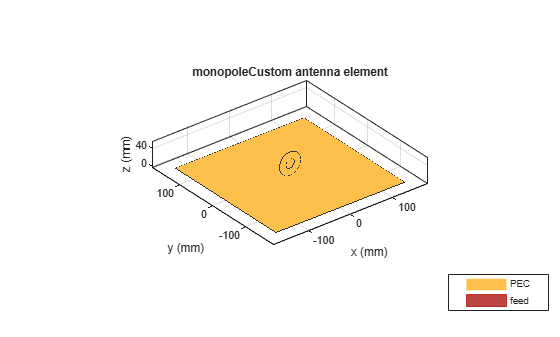#### Create Antenna Mesh

Mesh the antenna manually by using at least 10 elements per wavelength at `3 GHz` before running the analysis. Set the `MaxEdgeLength` to L`ambda/10`.

```figure; mesh(ant,"MaxEdgeLength",0.01)```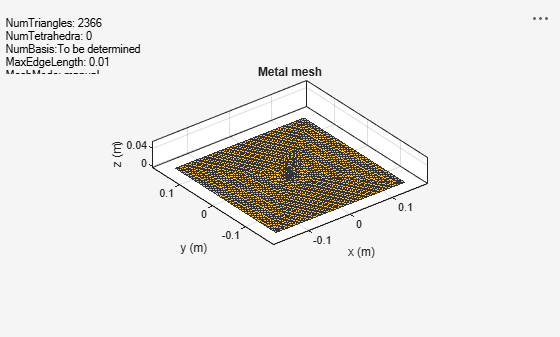#### Calculate VSWR

Calculate the voltage standing wave ratio (VSWR) of the annular ring planar monopole antenna to find the impedance bandwidth of the antenna. The VSWR is less than 2 in the the frequency range of `1.2 GHz` to `8.7 GHz`.

```figure; vswr(ant,(0.5:0.2:10)*1e9,50); ylim([1 5]);```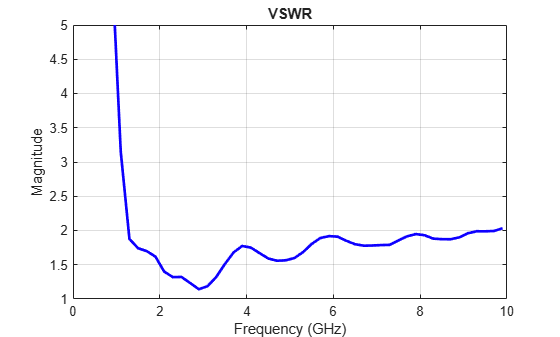Plot VSWR for different planar antennas where the` rSmall `is set to `5 mm, 10 mm, 15 mm and 20 mm.`

```load annularRingVSWR.mat % load data from annularRingVSWR.mat file figure; plot(freq,r5,'LineStyle',"-","Color",'r','LineWidth',1.5); grid on; hold on; plot(freq,r10,'LineStyle',"-.","Color",'k','LineWidth',1.5); plot(freq,r15,'LineStyle',"--","Color",'b','LineWidth',1.5); plot(freq,r20,'LineStyle',":","Color",'g','LineWidth',1.5); ylim([1 5]); xlabel('Frequency (GHz)'); ylabel('Magnitude'); title('VSWR'); legend('rSmall = 5mm', 'rSmall = 10mm', 'rSmall = 15mm', 'rSmall = 20mm')```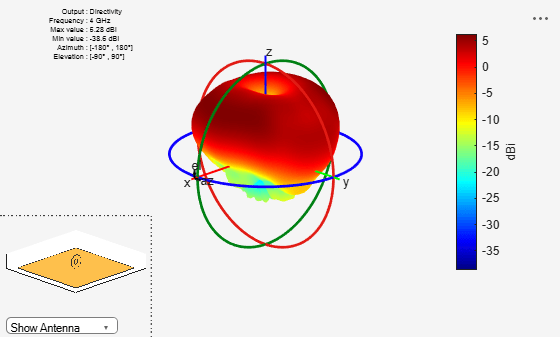You can see that as the value of `rSmall` increases from `5 mm` to `10 mm,` there is change in the upper band edge frequency from 9.7 GHz to 8.7 GHz. As this increases further, the antenna becomes a multiband antenna instead of a wideband antenna. In all these cases, the lower band edge frequency is the same. In this example, the `rSmall` or the innerCircle radius value is set to `10 mm`.

#### Plot Antenna Radiation Pattern

Analyze the radiation pattern of the antenna is at `4 GHz`.

`figure; pattern(ant,4e9);`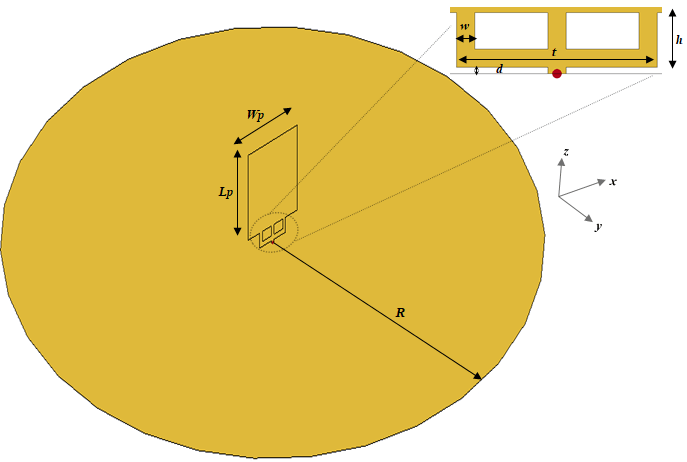At `4 GHz`, the annular ring monopole antenna exhibits typical omnidirectional monoplanar radiation pattern with a maximum gain of `6.23 dBi`. The simulation results agree with the results presented in .

### Square Planar Monopole Antenna with Trident-Shaped Feeding Strip

This figure shows the geometry and dimensions of a square planar antenna with the square plane has a side length of `34 mm. `The trident-shaped feeding strip parameters are: distance between the ground plane and the feeding strip (d) is `1 mm`, height of the feeding strip from d (h) is `4 mm`, the horizontal length of the feeding strip (t) is `18 mm`, and the width (w) of all the three strips that make the trident is `2 mm`. The radius of the ground plane (`R)` is `150 mm`. The idea of the trident-fed square monopole is taken from , but in this example uses a circular ground plane.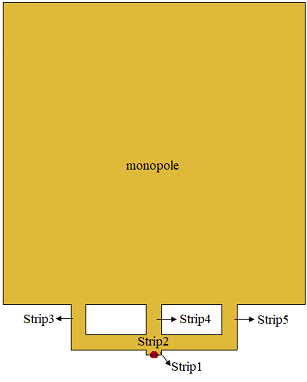The radiator comprises of a trident-shaped feeding strip and square monopole. Create the trident-shaped feeding strip object, Feed, by adding the `Strip1`, `Strip2`, `Strip3`, `Strip4` and `Strip5 `shapes. To create radiator, add the `Feed` with `monopole `shape.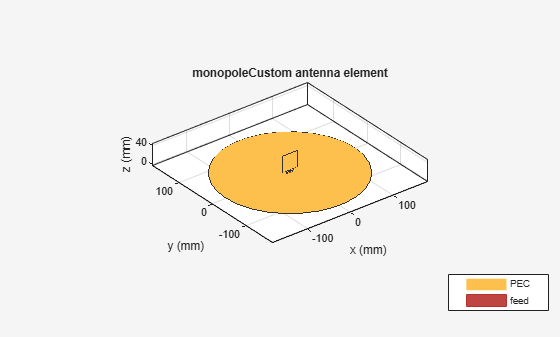```Lp = 34e-3; Wp = 34e-3; h = 4e-3; d = 1e-3; w = 2e-3; t = 18e-3; Strip1 = antenna.Rectangle("Length",w,"Width",d,"Center",[0 d/2]); Strip2 = antenna.Rectangle("Length",t,"Width",w,"Center",[0 d+w/2]); Strip3 = antenna.Rectangle("Length",w,"Width",h,"Center",[-(t/2-w/2) d+w+h/2]); Strip4 = antenna.Rectangle("Length",w,"Width",h,"Center",[0 d+w+h/2]); Strip5 = antenna.Rectangle("Length",w,"Width",h,"Center",[t/2-w/2 d+w+h/2]); Feed = Strip1+Strip2+Strip3+Strip4+Strip5; monopole = antenna.Rectangle("Length",Lp,"Width",Wp,"Center",[0 d+w+h+Wp/2]); radiator = Feed + monopole;```

#### Create Ground Plane

Create the circular ground plane using the `antenna.Circle` function with radius of `150 mm`.

```R = 150e-3; groundPlane = antenna.Circle("Radius",R);```

#### Create Planar Monopole

To create a trident-fed square planar monopole antenna, use the `monopoleCustom` object.

```ant = monopoleCustom ("Radiator",radiator,"GroundPlane",groundPlane); show(ant)```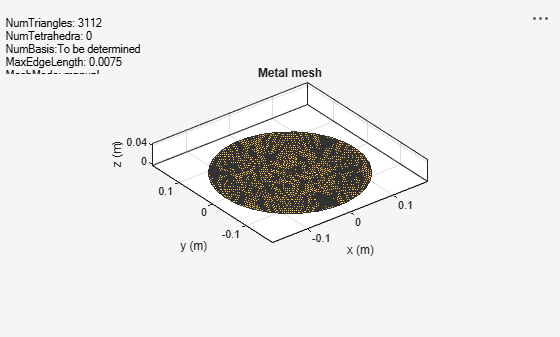#### Meshing antenna

Mesh the antenna manually by using at least 10 elements per wavelength at `4 GHz` before running the analysis. Set the `MaxEdgeLength` to L`ambda/10`.

```figure; mesh(ant,"MaxEdgeLength",0.0075);```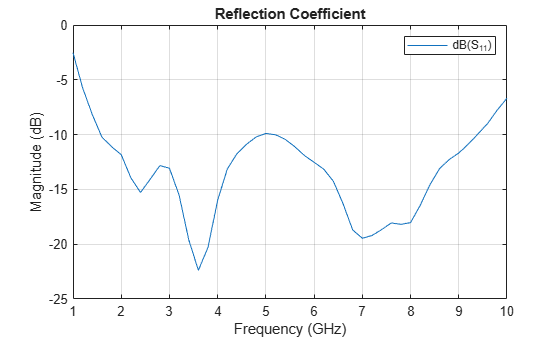#### Calculate Reflection Coefficient

Calculate the reflection coefficient of the planar monopole antenna to find the impedance bandwidth of the antenna. The reflection coefficient is less than -10 dB in the frequency range 1.6 GHz to 9.3 GHz.

```freq = (1:0.2:10)*1e9; s = sparameters(ant,freq); figure; rfplot(s); title('Reflection Coefficient');```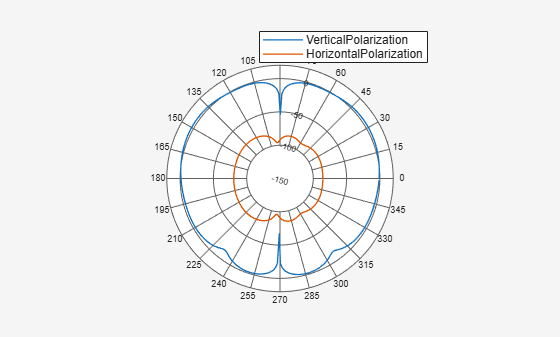#### Plot Radiation Pattern

Analyzing the radiation pattern of the antenna at `2 GHz, 6 GHz,` and `9 GHz `shows the vertical polarization as stable in the entire range of frequencies. But as the frequency increases, there is an increase in the horizontal polarization component.

```pV_1 = pattern (ant, 2e9, 0, 0:1:360,'Polarization','V'); pH_1 = pattern (ant, 2e9, 0, 0:1:360,'Polarization','H'); figure; polarpattern(pV_1,'MagnitudeLim',[-150 20]); hold on; polarpattern(pH_1,'MagnitudeLim',[-150 20]); legend VerticalPolarization HorizontalPolarization;```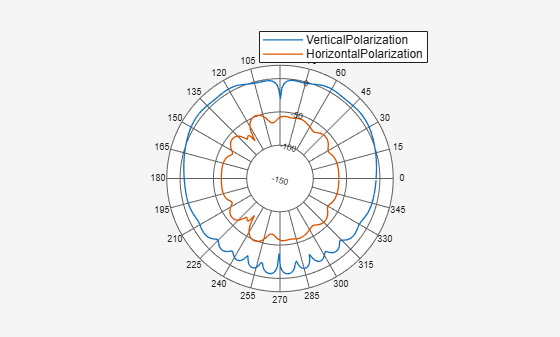```pV_2=pattern (ant, 6e9, 0, 0:1:360,'Polarization','V'); pH_2=pattern (ant, 6e9, 0, 0:1:360,'Polarization','H'); figure; polarpattern(pV_2,'MagnitudeLim',[-150 20]); hold on; polarpattern(pH_2,'MagnitudeLim',[-150 20]); legend VerticalPolarization HorizontalPolarization;``````pV_3 = pattern (ant, 9e9, 0, 0:1:360,'Polarization','V'); pH_3 = pattern (ant, 9e9, 0, 0:1:360,'Polarization','H'); figure; polarpattern(pV_3,'MagnitudeLim',[-150 20]); hold on; polarpattern(pH_3,'MagnitudeLim',[-150 20]); legend VerticalPolarization HorizontalPolarization;```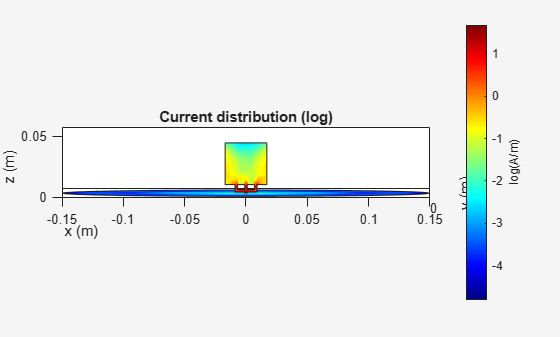#### View Current Distribution

The current distribution of the antenna is analyzed at `2.5 GHz`. The trident-shaped feeding structure results in uniform current distribution in the lower part of the square monopole.

```current(ant,2.5e9,'scale','log'); view(0,1)```#### Conclusion

Planar monopole antennas have simple geometry, and you can define their radiators and the ground planes in variety of different shapes. These antennas offer ultrawide impedance bandwidth with an azimuthal radiation pattern that is nearly omnidirectional.

#### Reference

 Ammann, M. J. Chen, Z. N. Chia, M. Y. W. and See, T. S. P. . “Annular Planar Monopole Antennas.” IEE Proceedings - Microwaves, Antennas and Propagation, Vol. 149, No. 4, August 2002, pp. 200–203.

 Kin-Lu Wong, Chih-Hsien Wu and Saou-Wen Su, "Ultrawide-band square planar metal-plate monopole antenna with a trident-shaped feeding strip," IEEE Transactions on Antennas and Propagation, Vol. 53, No. 4, April 2005, pp. 1262-1269.